StatLect

# Joint probability density function

The joint probability density function (joint pdf) is a function used to characterize the probability distribution of several continuous random variables, which together form a continuous random vector.## Introduction

It is a multivariate generalization of the probability density function (pdf), which describes the distribution of a single continuous variable.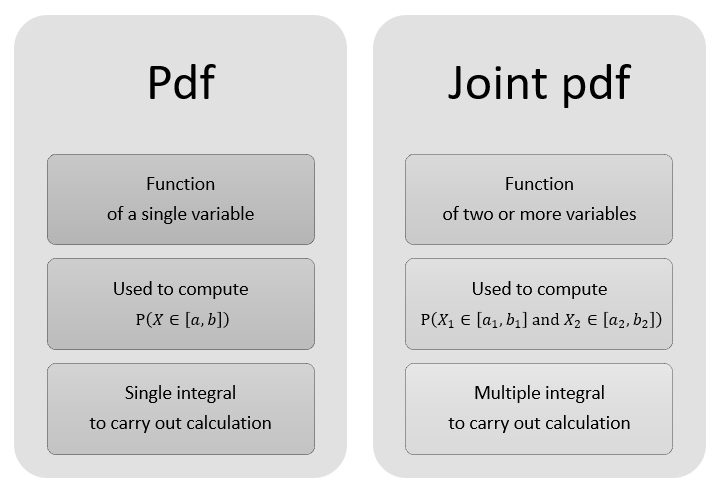The generalization works as follows:

• the integral of the density of a continuous variable over an interval is equal to the probability that the variable will belong to that interval;

• the multiple integral of the joint density of a continuous vector over a given set is equal to the probability that the random vector will belong to that set.

Do not worry if you do not know how to compute multiple integrals. We will explain them below!

## Definition

The following is a formal definition.

Definition Letbe a continuous random vector formed by the random variables. The joint probability density function ofis a functionsuch that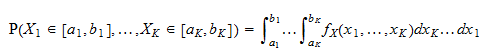for any choice of the intervalsNote that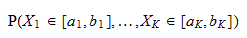is the probability that the following conditions are simultaneously satisfied:

1. the first entry of the vectorbelongs to the interval;

2. the second entry of the vectorbelongs to the interval;

3. and so on.

The notationmeans that the multiple integral is computed along all thecoordinates (see below for more details).

We often denote the joint pdf bywhich is equivalent towhereare theentries of the vector.

## How to work out the multiple integral

Multiple integrals are relatively easy to work out.

Take, for example, a double integral:wheretakes values in the intervalandin the interval.

The integral is computed in two steps:

1. in the first step, we compute the inner integral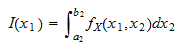which gives a function of(disappears because it has been integrated out);

2. in the second step, we compute the integral of, that is,If there are more than two integrals, the procedure is similar: we work out the integrals one by one, starting from the innermost one.

## Examples

Here are some examples.

### Example 1

Consider the joint pdf of two variables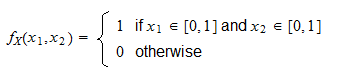In other words, the joint pdf is equal toif both entries of the vector belong to the intervaland it is equal tootherwise.

Suppose that we need to compute the probability that both entries will be less than or equal to.

This probability can be computed as a double integral: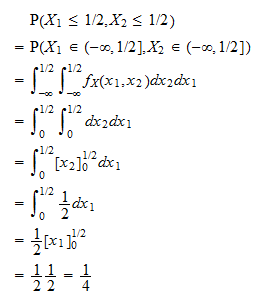### Example 2

Consider two variables having joint probability density function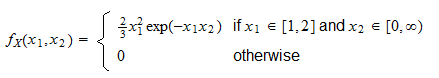Suppose that we want to calculate the probability thatis greater than or equal toand at the same timeis less than or equal to.

This can be accomplished as follows: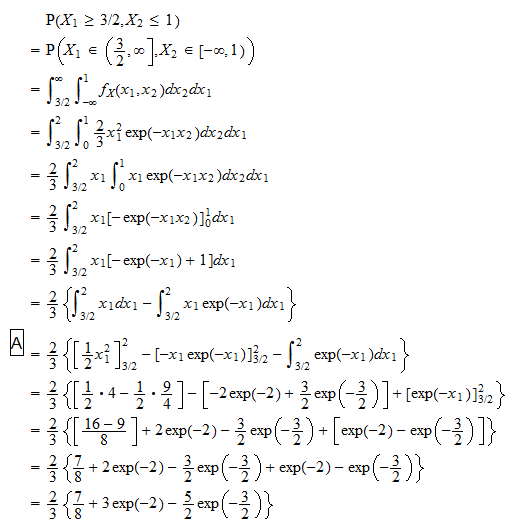where in stepwe have performed an integration by parts.

## Joint and marginal density

One of the entries of a continuous random vector, when considered in isolation, can be described by its probability density function, which is called marginal density.

The joint density can be used to derive the marginal density. How to do this is explained in the glossary entry about the marginal density function.

## More details

Joint probability density functions are discussed in more detail in the lecture on Random vectors.

Previous entry: Joint distribution function

Next entry: Joint probability mass function